# WIZnet Controlled Robotic Arm

Control your robotic arm from long distance using Ethernet.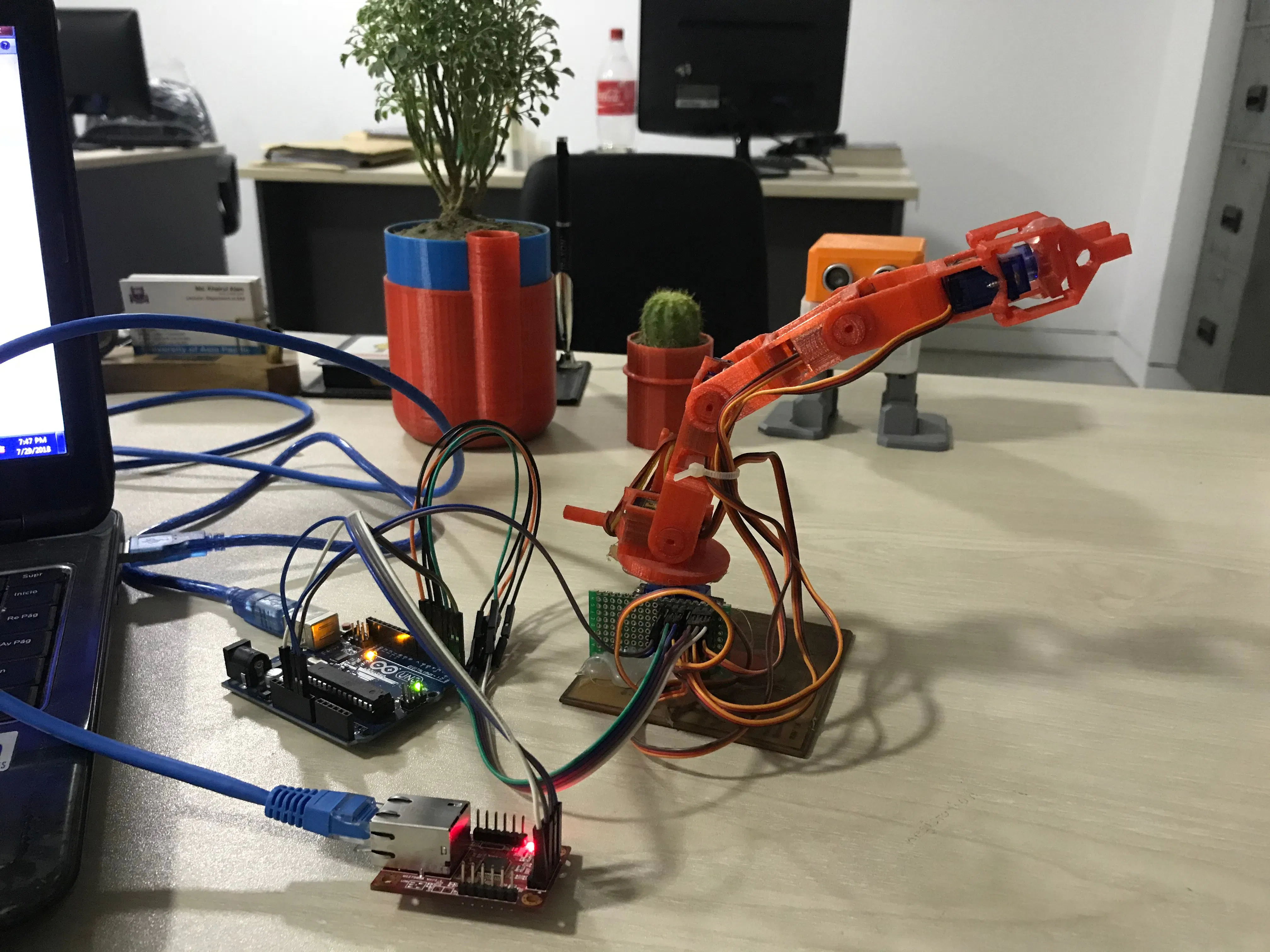## Things used in this project

### Hardware components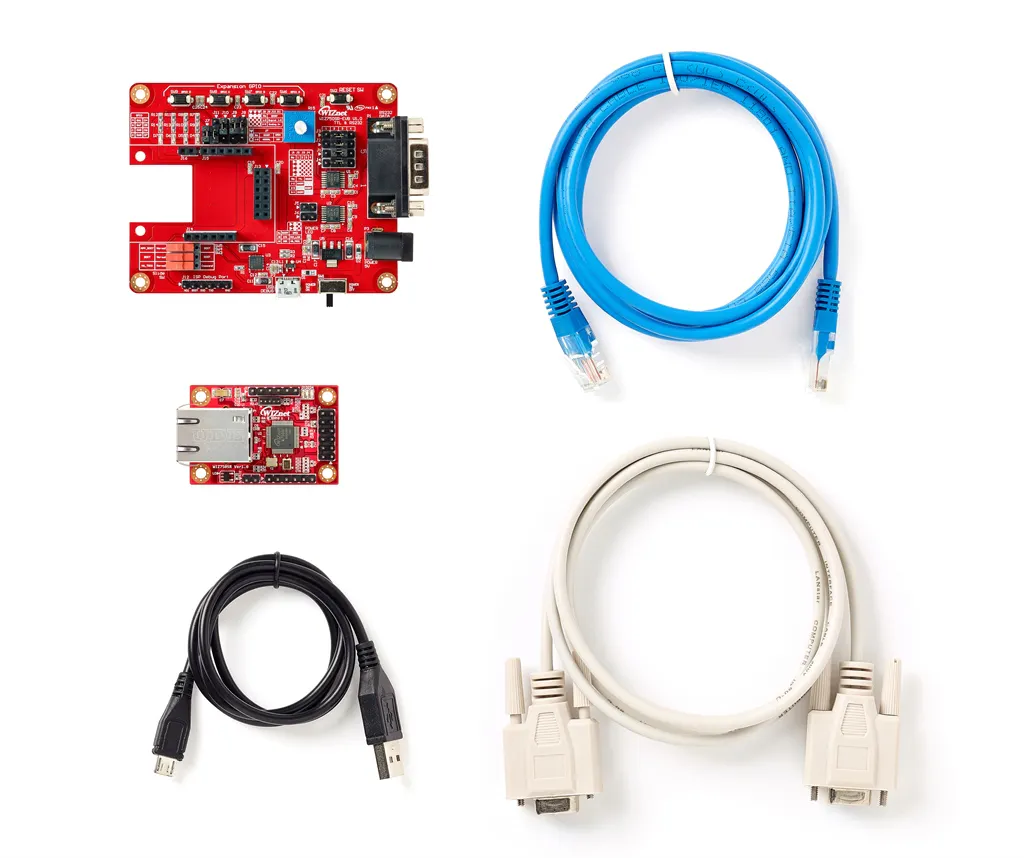WIZnet WIZ750SR-TTL-EVB Kit
×1Arduino UNO & Genuino UNO
×1
 Generic Robotic Arm
×1

### Software apps and online servicesArduino IDEThe Processing Foundation Processing

## Schematics

### Connection of servo motors to Arduino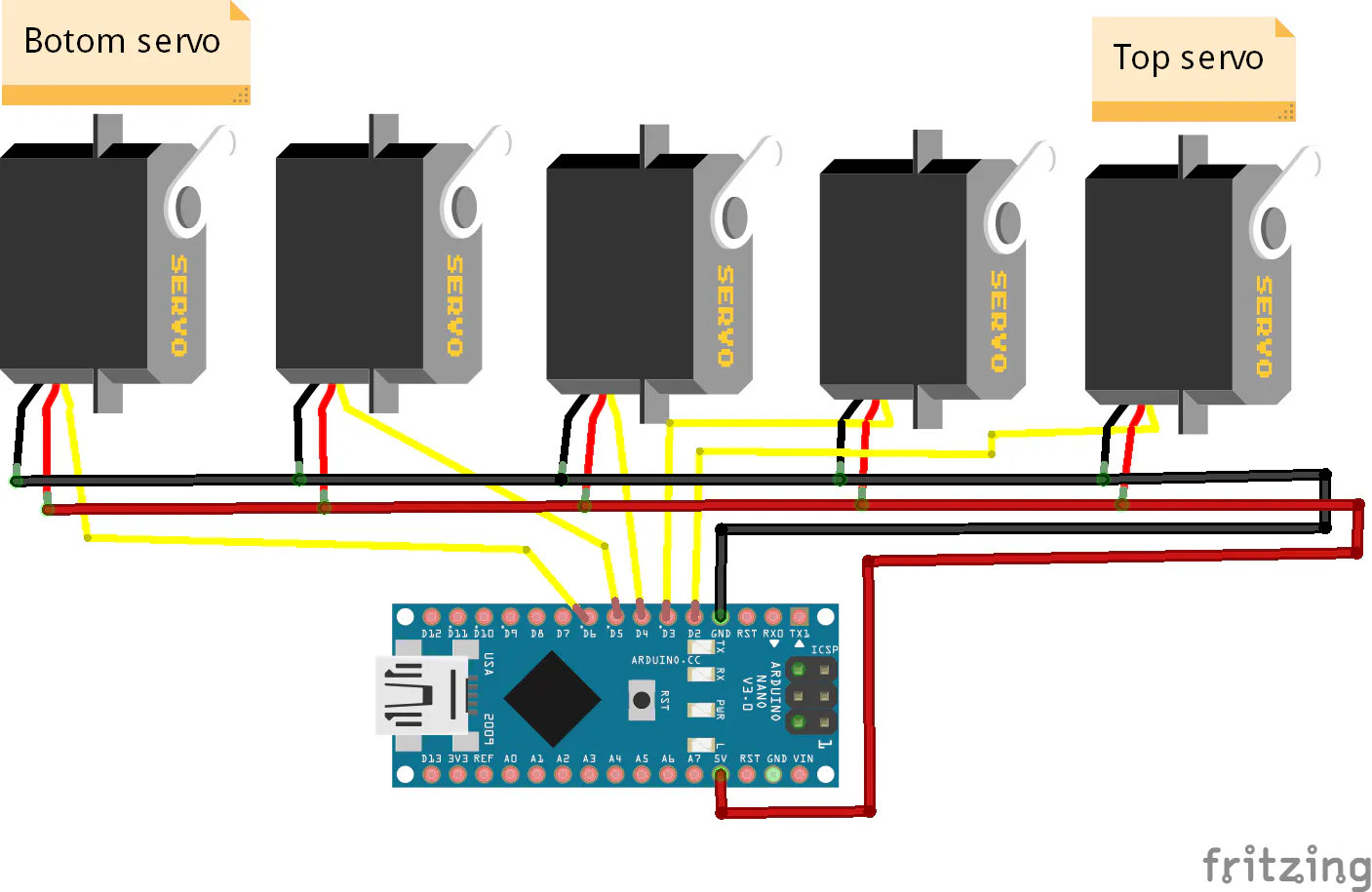### Graphical Interface of Desktop Application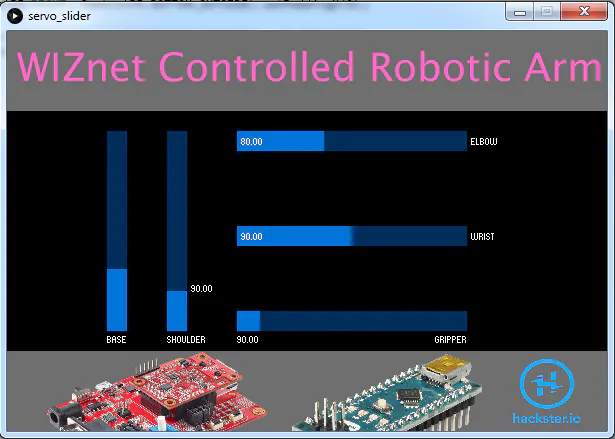### Virtual COM Port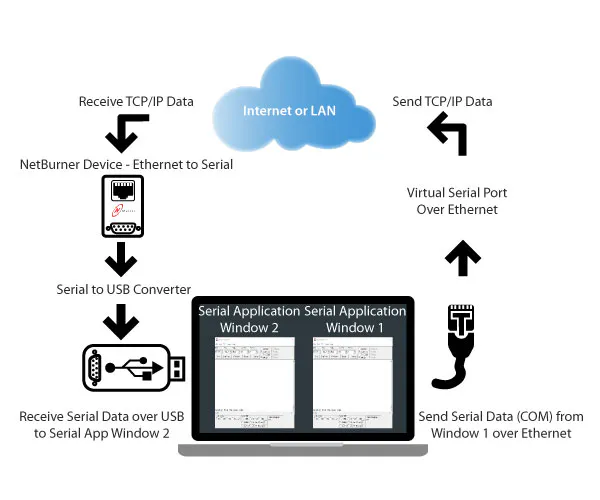### WIZ750SR Pinout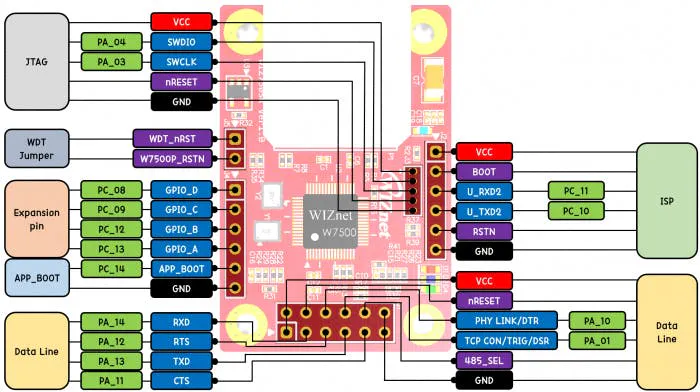## Code

### Code for Desktop Application

Processing
The app was developed using processing. Before running the program add the ControlP5 library from the link: http://www.sojamo.de/libraries/controlP5/
```/**
Wiznet controlled robotic arm
Author: Md. Khairul Alam
*/
import controlP5.*;
import processing.serial.*; //import the Serial library

Serial myPort;  //the Serial port object
PImage img;
PImage img1;
PImage img2;

ControlP5 cp5;
int myColor = color(0,0,0);
int topColor = color(110,110,110);
int bottomColor = color(110,110,110);
int tColor = color(0,200,0);

void setup() {
size(600,400);
noStroke();

cp5 = new ControlP5(this);

// create five slider
.setPosition(100,100)
.setSize(20,200)
.setRange(0,180)
.setValue(90)
;

.setPosition(160,100)
.setSize(20,200)
.setRange(70,170)
.setValue(90)
;

.setPosition(230,100)
.setSize(230,20)
.setRange(25,170)
.setValue(80)

;

.setPosition(230,195)
.setSize(230,20)
.setRange(0,180)
.setValue(90)
;

.setPosition(230,280)
.setSize(230,20)
.setRange(80,180)
.setValue(90)
;

//myPort = new Serial(this, Serial.list(), 9600);
myPort = new Serial(this, "COM20", 115200);
myPort.bufferUntil('\n');

}

void draw() {
background(165,165,165);

fill(topColor);
rect(0,0,width,100);

textSize(39);
fill(255, 102, 200);
text("WIZnet Controlled Robotic Arm", 10, 50);

fill(myColor);
rect(0,80,width,240);

fill(bottomColor);
rect(0,320,width,100);
image(img, 0, 280, width/2, height/2);
image(img1, 280, 320, width/3, height/3);
image(img2, 490, 320, width/6, height/5);
}

void controlEvent(ControlEvent theEvent) {
/* events triggered by controllers are automatically forwarded to
the controlEvent method. by checking the name of a controller one can
distinguish which of the controllers has been changed.
*/

/* check if the event is from a controller otherwise you'll get an error
when clicking other interface elements like Radiobutton that don't support
the controller() methods
*/

if(theEvent.isController()) {

print("control event from : "+theEvent.getController().getName());
float posValue = theEvent.getController().getValue();
println(", value : "+posValue);
int temp = int(posValue);

if(theEvent.getController().getName()=="BASE") {
println("Base is moveing");
String pos = str(temp);
myPort.write(pos);          //send position
myPort.write('\n');
delay(100);
}

if(theEvent.getController().getName()=="SHOULDER") {
println("Shoulder is moveing");
String pos = str(temp+180);
myPort.write(pos);
myPort.write('\n');
delay(100);
}

if(theEvent.getController().getName()=="ELBOW") {
println("Elbow is moveing");
String pos = str(temp+360);
myPort.write(pos);
myPort.write('\n');
delay(100);
}

if(theEvent.getController().getName()=="WRIST") {
println("Wrist is moveing");
String pos = str(temp+540);
myPort.write(pos);
myPort.write('\n');
delay(100);
}

if(theEvent.getController().getName()=="GRIPPER") {
println("Gripper is moveing");
String pos = str(temp+720);
myPort.write(pos);
myPort.write('\n');
delay(100);
}

}
}

void serialEvent (Serial myPort) {
// get the byte:
// print it:
println(inByte);
}
```

### Arduino Sketch for controlling the Arm

Arduino
This code was developed to control five servo based on the data received from serial port.
```/*************************************
* Author: Md. Khairul Alam
* This code is for controlling a robotic arm containing 5 servos
*/

#include <Servo.h>

Servo servo1, servo2, servo3, servo4, servo5;
String inputString = "";         // a string to hold incoming data
boolean stringComplete = false;  // whether the string is complete

int gripper = 2;
int wrist = 3;
int elbow = 4;
int shoulder = 5;
int base = 6;

void setup() {
// put your setup code here, to run once:
Serial.begin(115200);
servo1.attach(gripper);
servo2.attach(wrist);
servo3.attach(elbow);
servo4.attach(shoulder);
servo5.attach(base);
inputString.reserve(200);
}

void loop() {

if (stringComplete) {
int pos = inputString.toInt();
if(pos <= 180){//if received message = pos1
set_base(pos);
delay(20);
}
else if(pos>250 && pos <=350){
set_shoulder(pos-180);
delay(20);
}
else if(pos>385 && pos <=530){
set_elbow(pos-360);
delay(20);
}
else if(pos>540 && pos <=720){
set_wrist(pos-540);
delay(20);
}
else if(pos>800 && pos <=900){
set_gripper(pos-720);
delay(20);
}
// clear the string:
inputString = "";
stringComplete = false;
}

}

void servo_rotate(int num, int value){
switch(num){
case 1:
servo1.write(value);
break;

case 2:
servo2.write(value);
break;

case 3:
servo3.write(value);
break;

case 4:
servo4.write(value);
break;

case 5:
servo5.write(value);
break;
}
}

//this function grip an object
//this function drive servo 5 to set x position in angle
void set_base(int pos){
if(pos>previous_pos){
for(int i=previous_pos; i<pos; i+=2){
servo_rotate(5, i);
//delay(25);
delay(5);
}
}
if(pos<previous_pos){
for(int i=previous_pos; i>pos; i-=2){
servo_rotate(5, i);
//delay(25);
delay(5);
}
}
}
//set sevo 3 position
void set_elbow(int pos){
if(pos>previous_pos){
for(int i=previous_pos; i<pos; i+=2){
servo_rotate(3, i);
delay(5);
}
}
if(pos<previous_pos){
for(int i=previous_pos; i>pos; i-=2){
servo_rotate(3, i);
delay(5);
}
}
}
//set sevo 2 position
void set_wrist(int pos){
if(pos>previous_pos){
for(int i=previous_pos; i<pos; i+=2){
servo_rotate(2, i);
delay(5);
}
}
if(pos<previous_pos){
for(int i=previous_pos; i>pos; i-=2){
servo_rotate(2, i);
delay(5);
}
}
}

//set sevo 1 position
void set_gripper(int pos){
if(pos>previous_pos){
for(int i=previous_pos; i<pos; i+=2){
servo_rotate(1, i);
delay(5);
}
}
if(pos<previous_pos){
for(int i=previous_pos; i>pos; i-=2){
servo_rotate(1, i);
delay(5);
}
}
}
//set sevo 4 position
void set_shoulder(int pos){
if(pos>previous_pos){
for(int i=previous_pos; i<pos; i+=2){
servo_rotate(4, i);
delay(5);
}
}
if(pos<previous_pos){
for(int i=previous_pos; i>pos; i-=2){
servo_rotate(4, i);
delay(5);
}
}
}

void serialEvent() {
while (Serial.available()) {
// get the new byte:
// if the incoming character is a newline, set a flag
// so the main loop can do something about it:
if (inChar == '\n') {
stringComplete = true;
}
else
// add it to the inputString:
inputString += inChar;
}
}
```

## Credits

### Md. Khairul Alam

43 projects • 400 followers
Engineer, developer, maker & hacker. Currently working as a faculty at the University of Asia Pacific, Dhaka, Bangladesh.